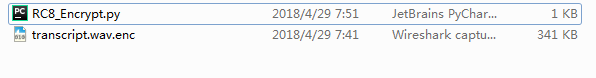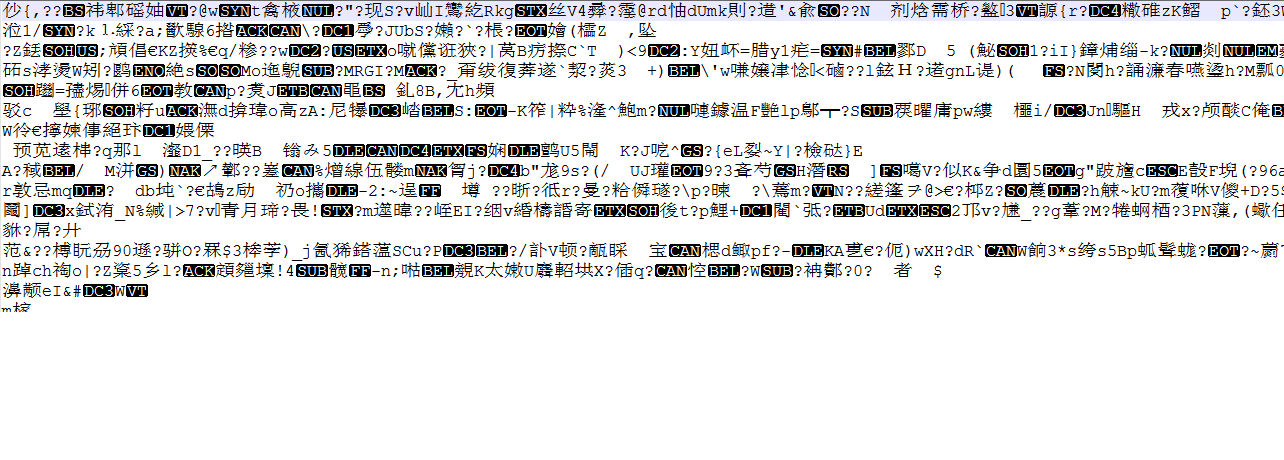# 1.题目分析A recent transcript suspected to contain incriminating conversations between the officials in charge of the Mars colonization plan has leaked but is encrypted with the latest top-secret encryption algorithm: RC8! You’ve recovered the source code to their new encryption algorithm, but the key and seed value are missing. Find a weakness in the scheme and recover the transcript.

#!/usr/bin/env python3
import sys

def rc8(state, key, n):
'''
Top Secret RC8 Stream Cipher
'''
while (n > 0):
yield state & 0xff
for _ in range(8):
c, s = key, state
b = 0
while c:
b ^= c & 1 * s & 1
c >>= 1 ; s >>= 1
state = state >> 1 | b << 63
n -= 1

def main():
seed, key = ?, ? # Missing

with open(sys.argv, 'rb') as fin:

for i,x in enumerate(rc8(seed, key, len(data))):
data[i] ^= x

with open(sys.argv + '.enc', 'wb') as fout:
fout.write(data)

if __name__ == "__main__":
main()

for _ in range(8):
c, s = key, state
b = 0
while c:
b ^= c & 1 * s & 1
c >>= 1 ; s >>= 1
state = state >> 1 | b << 63

c=c0c1cn1 c = c 0 c 1 ⋯ c n − 1 $c=c_{0}c_{1} \cdots c_{n-1}$ s=s0s1sn1 s = s 0 s 1 ⋯ s n − 1 $s=s_{0}s_{1} \cdots s_{n-1}$分别是c和s的二进制表示，根据对上面代码的分析我们不难得到下面式子：
$\qquad$ $\qquad$ b=(c0 b = ( c 0 $b=(c_{0}$& s0) s 0 ) $s_{0})$^ (c1 ( c 1 $(c_{1}$& s1) s 1 ) $s_{1})$^ $\cdots$^ (cn1 ( c n − 1 $(c_{n-1}$& sn1) s n − 1 ) $s_{n-1})$

b(c0×s0+c1×s1++cn1×sn1)mod2 b ≡ ( c 0 × s 0 + c 1 × s 1 + ⋯ + c n − 1 × s n − 1 ) m o d 21-44“RIFF”表示文件”RIFF”文件
5-84Uint32文件长度-8
9-124“WAVE”文件类型头，表示一个”WAVE”文件
13-164“fmt “格式表示符

>>>import os
>>>hex(os.path.getsize("transcript.wav.enc")-8)
>>>'0x55030L'

52 49 46 46 30 50 05 00 57 41 56 45 66 6D 74 20

81 A3 7B 2C CD 36 EB A0 08 B5 74 DB AF B4 74 E6

D3 EA 3D 6A FD 66 EE 30 5F F4 22 9E C9 D9 00 C6

# 2.破解加密

D3 EA 3D 6A FD 66 EE 30 5F F4 22 9E C9 D9 00 C6

b0=(c0×s0+c1×s1++cn1×sn1)mod2 b 0 = ( c 0 × s 0 + c 1 × s 1 + ⋯ + c n − 1 × s n − 1 ) m o d 2

b1=(c0×b0+c1×s0++cn1×sn2)mod2 b 1 = ( c 0 × b 0 + c 1 × s 0 + ⋯ + c n − 1 × s n − 2 ) m o d 2

s0b0bn2s1s0bn3s2s1bn4sn1sn2s0c0c1cn1b0b1bn1mod2 ( s 0 s 1 s 2 ⋯ s n − 1 b 0 s 0 s 1 ⋯ s n − 2 ⋮ ⋮ ⋮ ⋯ ⋮ b n − 2 b n − 3 b n − 4 ⋯ s 0 ) ( c 0 c 1 ⋮ c n − 1 ) ≡ ( b 0 b 1 ⋮ b n − 1 ) m o d 2

sage\$ sage sol_rc8.sage [+] Seed: 3525868796872878803 [+] Key: 36028806108921863

# 3.总结

• 了解将0和1的与运算及异或运算变为乘法和模加运算
• 知道wav格式的文件头知识
• 分析代码得到方程后，知道如何利用强大的数学软件sage解方程

04-19188610-2630万+
08-23
09-17904
04-017203
09-171万+
05-291181
05-237590
02-132238
12-068885
05-286412
05-282340
07-2881
11-111249
06-125747
07-1087
05-124万+点击重新获取扫码支付余额充值